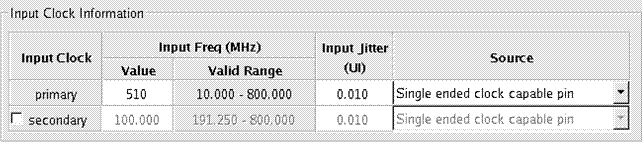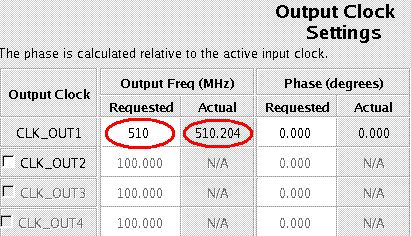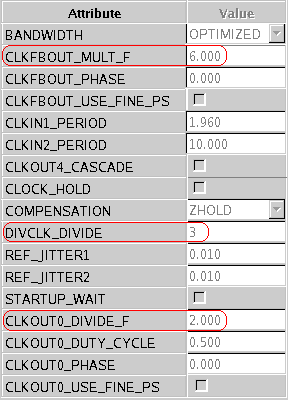# AR# 34937

## Description

When using the Clocking Wizard and the Output clock frequency as the input Clock, sometimes the Request and Actual Frequencies are different in the Wizard. Why?

## Solution

1) The Clocking Wizard performs the calculations to find M, D and CLKOUTx_DIVIDE rounding to 3 decimal places, which is consistent with TRCE. This can cause minor rounding error.

For example, if the input frequency is 61.44MHz and the request CLKOUT frequency = 44.8MHz, this will result in an Actual CLKOUT frequency =44.807MHz

The first step the Wizard does is convert the input frequency to period and round to 3 decimal places.

1000/61.44 = 16.276041666666666666666666666667 =~ 16.276
VCO = (1000/16.276) * 33 / 2 = 1013.7625952322437945441140329319 =~ 1013.763
CLKOUT frequency =1013.763/22.625 = 44.807204419889502762430939226519 =~ 44.807

CLKFBOUT_MULT_F = 33, CLKOUT0_DIVIDE_F = 22.625, DIVCLK_DIVIDE = 2

2) The rounding error may also cause a minor discrepancy between the displayed Actual frequency and what is output.

For example if you input 510MHz andrequest 510MHz.

Here the CLKIN is entered as 510 MHz:When selecting 510 MHz as the output Frequency, you can see that instead of getting 510 MHz, the Actual is listed as 510.204 MHz:

The reason for this is how the calculations are done; they only use 3 decimal places. The following is how the 510.204 MHz is created:Computed Clock Period = 1/510 MHz = 1.960784314ns with 3 decimal places = 1.960 ns Computed Output Frequency = 1/1.960nS = 510.204 MHz

In Hardware, the output frequency will be 510 MHz as Requested. In order to verify this, the output frequency can be calculated using the settings on page 4 of the wizard:So CLKOUT0 = ((CLKIN * CLKFBOUT_MULT_F) / DIVCLK_DIVIDE ) / CLKOUT0_DIVIDE_F

((510 MHz * 6) / 3 ) / 2 = 510 MHz.